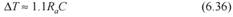Create an Account

Home / Questions / Determine the exact pulse width as a function of the resistance and capacitance in a one s...

Determine the exact pulse width as a function of the resistance and capacitance in a one shot circuit see Equation

Determine the exact pulse width as a function of the resistance and capacitance in a one-shot circuit (see Equation 6.36).Jul 26 2020 View more View LessSubscribe To Get Solution